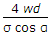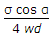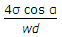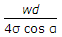# Mechanical Engineering - Hydraulics and Fluid Mechanics

### Exercise :: Hydraulics and Fluid Mechanics - Section 5

1.

A glass tube of small diameter (d) is dipped in fluid. The height of rise or fall in the tube given by (where w = Specific weight of liquid, α = Angle of contact of the liquid surface, and σ = Surface tension)

 A.B.C.D.Explanation:

No answer description available for this question. Let us discuss.

2.

An internal mouthpiece is said to be running free if the length of the mouthpiece is __________ the diameter of the orifice.

 A. less than twice B. more than twice C. less than three times D. more than three times

Explanation:

No answer description available for this question. Let us discuss.

3.

The discharge over a rectangular notch is

 A. inversely proportional to H3/2 B. directly proportional to H3/2 C. inversely proportional to H5/2 D. directly proportional to H5/2

Explanation:

No answer description available for this question. Let us discuss.

4.

The volume of a fluid __________ as the pressure increases.

 A. remains same B. decreases C. increases

Explanation:

No answer description available for this question. Let us discuss.

5.

The pressure of the liquid flowing through the divergent portion of a venturimeter

 A. remains constant B. increases C. decreases D. depends upon mass of liquid